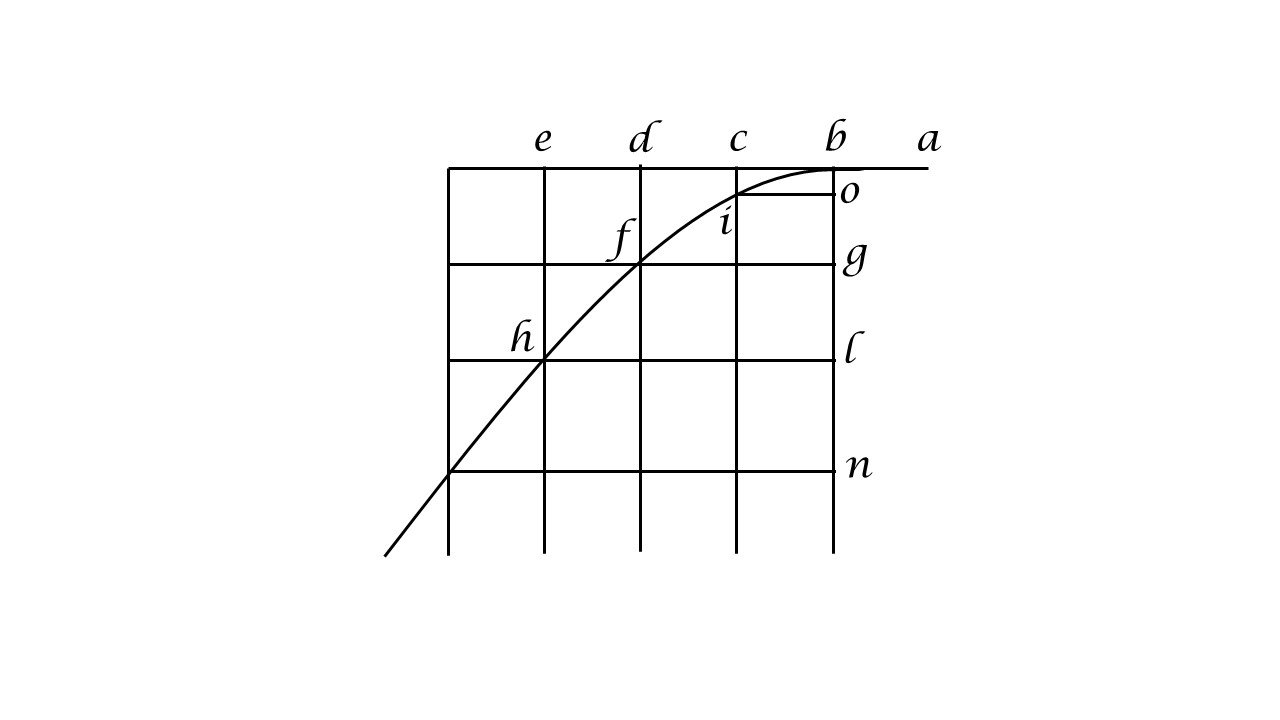iSoul In the beginning is reality.

# Ratios of length and duration

This post relates to others such as this.

Consider Galileo’s figure (see his Dialogues Concerning Two New Sciences, tr. Crew & De Salvio p.249 Fig. 108 or Drake’s translation p.221):A projectile moves with uniform velocity horizontally to the left and begins to descend at point b. Galileo used the sequence a-b-c-d-e to represent time and the sequence b-o-g-l-n to represent the height of the projectile above the Earth. The sequence b-i-f-h represents the parabolic path of the falling projectile.

Any uniform motion can serve as a reference motion. There are two uses of a reference variable: (i) as a parametric variable, or (ii) as a measurement variable. A parametric variable is an independent variable that provides ordered input for any dependent variable. A measurement variable is a variable that is dependent on the independent variable being measured. In the figure above the parametric variable is the time (duration) of the uniform motion on the horizontal axis, and the measurement variable is the height (length) of the uniform acceleration on the vertical axis.

Combine this with the two measures of motion, length and duration, and there are four possible cases: (1) independent duration variable with dependent length variable; (2) independent length variable with dependent duration variable; (3) independent length variable with dependent length variable; and (4) independent duration variable with dependent duration variable.

The figure above is an example of case (1). Its complement is case (2). Cases (3) and (4) include only one measure, length or duration, and so cannot express a rate of motion. Galileo expresses case (1) as a proportion between ratios of the variables at different times: s1 : s2 :: t12 : t22, which avoids combining different units in a single ratio, consistent with Eudoxian proportionality.

Consider case (2) in which the independent variable is length. This variable is a placeline for locating other motions, which is like a timeline except that it expresses an independent length as the order parameter. The dependent reference variable in this case is duration, which measures any independent variable, in this case projectile height. This could be expressed as a proportion between ratios of the variables at different times: t1 : t2 :: s12 : s22, avoiding different units in a single ratio.

Case (1) enables multiple length variables dependent on one independent variable, the timeline. Case (2) enables multiple duration variables dependent on one independent variable, the placeline. Rates of motion in case (1) are in units of the independent timeline, which is duration. Rates of motion in case (2) are in units of the independent placeline, which is length.